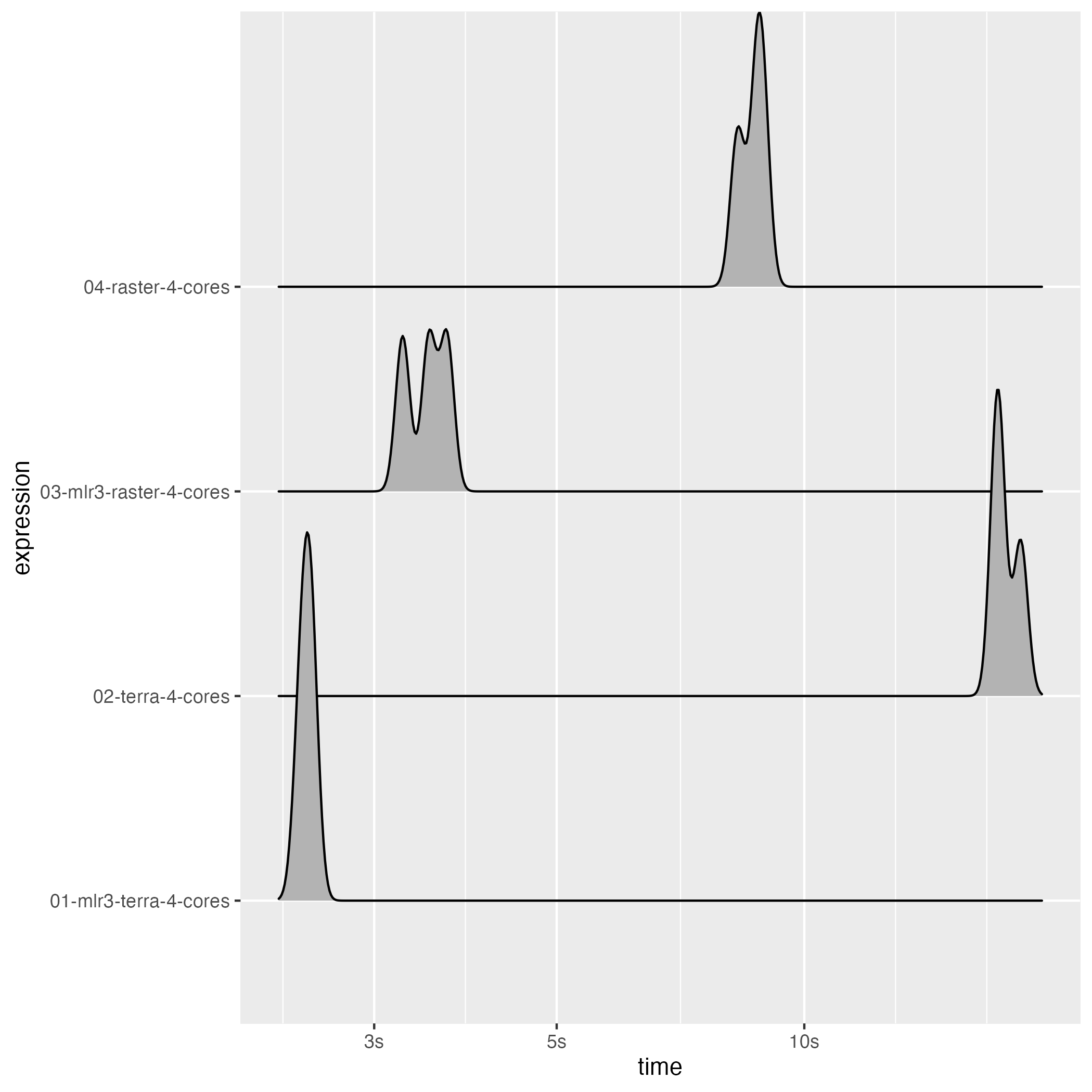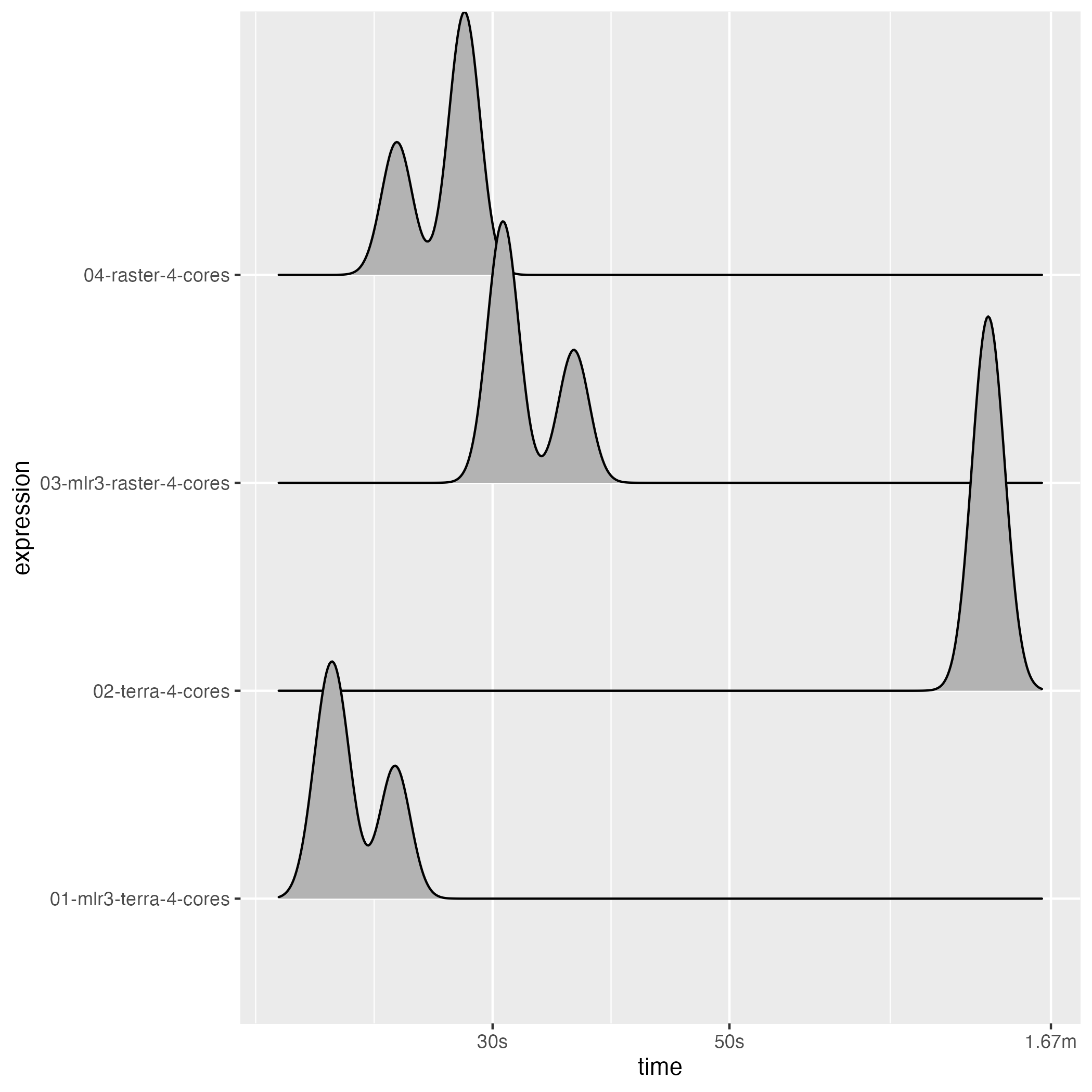# Benchmarking parallel predictions

This benchmark was run on a MacBook Pro 2020 with the following specs

• Intel i7-1068NG7 (8) @ 2.30GHz
• 32 GB DDR4 RAM

Note that the differences between the parallel and sequential timings will increase for larger objects as the overhead for starting the parallel workers and collecting the results will decrease.

It is not fully clear why the parallel approach of the {terra} package is slow than its sequential counterpart but it might relate to the single-core performance of the machine the benchmark was run on in combination with the overhead associated with starting the parallel cluster the way it is done in the {terra} package.

library(mlr3)
library(mlr3spatial)
library(future)
library(bench)
library(stars)
#> Linking to GEOS 3.9.1, GDAL 3.3.1, PROJ 8.1.0
library(rpart)

## Small files

• ~ 250k values
• 48 MB on disk
# SpatRaster demo stack
stack_terra = demo_stack_spatraster(50)
value = data.table::data.table(ID = c(0, 1), y = c("negative", "positive"))
terra::setCats(stack_terra, layer = "y", value = value)
colnames = names(stack_terra)
file_terra = tempfile("terra", fileext = ".tif")
terra::writeRaster(stack_terra, file_terra)

# RasterBrick demo stack
stack_raster = demo_stack_rasterbrick(50)
colnames_raster = names(stack_raster)
file_raster = tempfile("raster", fileext = ".tif")
raster::writeRaster(stack_raster, file_raster)
#> Warning in .gd_SetProject(object, ...): NOT UPDATED FOR PROJ >= 6

stack_terra = terra::rast(file_terra)
backend_terra = DataBackendRaster$new(stack_terra) task_terra = as_task_regr(backend_terra, target = "x_1") stack_raster = raster::brick(file_raster) names(stack_raster) = colnames_raster backend_raster = DataBackendRaster$new(stack_raster)
#> Warning in wkt(from): no wkt comment

# Train learners
set.seed(42)
row_ids = sample(1:task_terra$nrow, 50) learner_task_terra = lrn("regr.rpart") learner_task_terra$parallel_predict = TRUE
learner_task_terra$train(task_terra, row_ids = row_ids) learner_task_raster = lrn("regr.rpart") learner_task_terra$parallel_predict = TRUE
learner_task_raster$train(task_raster, row_ids = row_ids) # non-mlr3 models rpart_task_terra = rpart::rpart(x_1 ~ ., task_terra$data(rows = row_ids))
rpart_task_raster = rpart::rpart(x_1 ~ ., task_raster$data(rows = row_ids)) bm = bench::mark( "01-mlr3-terra-4-cores" = { plan(multicore, workers = 4) predict_spatial(task_terra, learner_task_terra, chunksize = 2000L) }, "02-terra-4-cores" = terra::predict(stack_terra, rpart_task_terra, cores = 4, cpkgs = "rpart"), "03-mlr3-raster-4-cores" = { plan(multicore, workers = 4) predict_spatial(task_raster, learner_task_raster, chunksize = 2000L, format = "raster") }, "04-raster-4-cores" = { library(raster) library(rpart) beginCluster(4, type = "PSOCK") clusterR(stack_raster, predict, args = list(model = rpart_task_raster)) }, check = FALSE, filter_gc = FALSE, min_iterations = 3, max_iterations = 3, memory = FALSE) #> Loading required package: sp #> #> Attaching package: 'raster' #> The following object is masked from 'package:future': #> #> values #> The following object is masked from 'package:mlr3': #> #> resample bm$itr/sec = NULL
bm$result = NULL bm$gc/sec = NULL
bm$memory = NULL bm$mem_alloc = NULL

print(bm)
#> # A tibble: 4 × 8
#>   expression                  min   median n_itr  n_gc total_time time           gc
#>   <bch:expr>             <bch:tm> <bch:tm> <int> <dbl>   <bch:tm> <list>         <list>
#> 1 01-mlr3-terra-4-cores     2.44s    2.49s     3    35      7.45s <bench_tm > <tibble [3 × 3]>
#> 2 02-terra-4-cores         17.16s   17.22s     3    26      52.7s <bench_tm > <tibble [3 × 3]>
#> 3 03-mlr3-raster-4-cores    3.25s     3.5s     3    34     10.42s <bench_tm > <tibble [3 × 3]>
#> 4 04-raster-4-cores          8.3s    8.74s     3     1     25.94s <bench_tm > <tibble [3 × 3]>
library(ggplot2)
autoplot(bm, type = "ridge")
#> Picking joint bandwidth of 0.00863## Large files

• ~ 25 Mio. values

• 485 MB on disk

# SpatRaster demo stack
stack_terra = demo_stack_spatraster(500)
value = data.table::data.table(ID = c(0, 1), y = c("negative", "positive"))
terra::setCats(stack_terra, layer = "y", value = value)
colnames = names(stack_terra)
file_terra = tempfile("terra", fileext = ".tif")
terra::writeRaster(stack_terra, file_terra)

# RasterBrick demo stack
stack_raster = demo_stack_rasterbrick(500)
colnames_raster = names(stack_raster)
file_raster = tempfile("raster", fileext = ".tif")
raster::writeRaster(stack_raster, file_raster)
#> Warning in .gd_SetProject(object, ...): NOT UPDATED FOR PROJ >= 6

stack_terra = terra::rast(file_terra)
backend_terra = DataBackendRaster$new(stack_terra) task_terra = as_task_regr(backend_terra, target = "x_1") stack_raster = raster::brick(file_raster) names(stack_raster) = colnames_raster backend_raster = DataBackendRaster$new(stack_raster)
#> Warning in wkt(from): no wkt comment

# Train learners
set.seed(42)
row_ids = sample(1:task_terra$nrow, 50) learner_task_terra = lrn("regr.rpart") learner_task_terra$parallel_predict = TRUE
learner_task_terra$train(task_terra, row_ids = row_ids) learner_task_raster = lrn("regr.rpart") learner_task_terra$parallel_predict = TRUE
learner_task_raster$train(task_raster, row_ids = row_ids) # non-mlr3 models rpart_task_terra = rpart::rpart(x_1 ~ ., task_terra$data(rows = row_ids))
rpart_task_raster = rpart::rpart(x_1 ~ ., task_raster$data(rows = row_ids)) bm = bench::mark( "01-mlr3-terra-4-cores" = { plan(multicore, workers = 4) predict_spatial(task_terra, learner_task_terra, chunksize = 2000L) }, "02-terra-4-cores" = terra::predict(stack_terra, rpart_task_terra, cores = 4, cpkgs = "rpart"), "03-mlr3-raster-4-cores" = { plan(multicore, workers = 4) predict_spatial(task_raster, learner_task_raster, chunksize = 2000L, format = "raster") }, "04-raster-4-cores" = { library(raster) library(rpart) beginCluster(4, type = "PSOCK") clusterR(stack_raster, predict, args = list(model = rpart_task_raster)) }, check = FALSE, filter_gc = FALSE, min_iterations = 3, max_iterations = 3, memory = FALSE) bm$itr/sec = NULL
bm$result = NULL bm$gc/sec = NULL
bm$memory = NULL bm$mem_alloc = NULL

print(bm)
#> # A tibble: 4 × 8
#>   expression                  min   median n_itr  n_gc total_time time           gc
#>   <bch:expr>             <bch:tm> <bch:tm> <int> <dbl>   <bch:tm> <list>         <list>
#> 1 01-mlr3-terra-4-cores    20.88s   21.55s     3   170      1.11m <bench_tm > <tibble [3 × 3]>
#> 2 02-terra-4-cores          1.44m    1.45m     3    27      4.37m <bench_tm > <tibble [3 × 3]>
#> 3 03-mlr3-raster-4-cores    30.5s   30.86s     3    38      1.62m <bench_tm > <tibble [3 × 3]>
#> 4 04-raster-4-cores         24.4s    28.1s     3     1      1.35m <bench_tm > <tibble [3 × 3]>
library(ggplot2)
autoplot(bm, type = "ridge")
#> Picking joint bandwidth of 0.0143